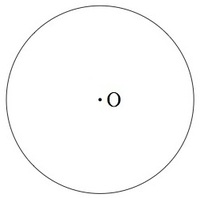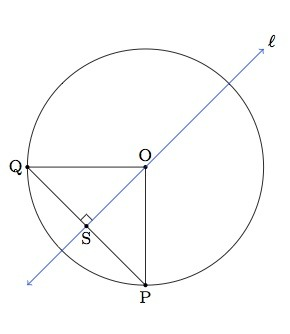# Symmetries of a circle

Alignments to Content Standards: G-CO.A

Suppose $C$ is a circle with center $O$ as pictured below:Find all lines of symmetry of the circle and explain why the list is complete.

## IM Commentary

The task 4.G Lines of symmetry for circles looks at lines of symmetry for a circle in a way appropriate for elementary school. Here these lines of symmetry are studied using the high school definition of reflections. Two different arguments are presented, the first using triangle congruence (alternatively, the Pythagorean theorem could be used here for an 8th grade solution) and another which uses rotations and reflections. If students do not think of the second solution the teacher may wish to suggest relating the different reflections of the circle via rotations: this helps to build visual intuition and also reveals the power of working in depth with rigid motions.

This task could be used for instruction or for assessment and should be done relatively early, shortly after students have been introduced to the formal definition of reflections using the notion of the perpendicular bisector of a line segment. The result is intuitively appealing, familiar, and gives a nice example of how to use the definition of reflections.

Students working on this task will engage in MP1 (Make Sense of Problems and Persevere in Solving Them) as they decide how to explain a familiar result using the definition of reflections. The second solution illustrates MP7 (Look For and Make Use of Structure) as all reflections are related to a single one by adding a rotation. This is also a good opportunity to engage in MP3 (Construct Viable Arguments and Critique the Reasoning of Others) if students work in groups and share their (possibly different) solutions.

## Solutions

Solution: 1 Triangle Congruence (or Pythagorean Theorem)

The lines of symmetry are precisely the lines through the center of the circle. There are two parts to this argument:

• Showing that the reflection of $C$ about a line $\ell$ containing $O$ sends $C$ to itself.
• Showing that the reflection of $C$ about a line $\ell$ not containing $O$ does not send $C$ to itself.

Let $r_{\ell}$ denote the reflection across the line $\ell$.

For the second part, if $O$ does not lie on $\ell$ then $r_\ell(O) \neq O$, so $r_\ell(C)\neq C$. (We can draw this conclusion more carefully by realizing that $O$ is the intersection of any two distinct diagonals of the circle, which must be preserved by the transformation).

Next we show that if $\ell$ contains the center $O$ of $C$, then $r_\ell(C) = C$. Suppose $P$ is a point on $C$. If $P$ also lies on $\ell$ then $r_\ell(P) = P$. Below is a sample picture in the case where $P$ does not lie on $\ell$:The reflection $Q = r_\ell(P)$ of $P$ has the property that $\ell$ is the perpendicular bisector of $\overline{PQ}$. So we know that $|SP| = |SQ|$. We also know that $|SO| = |SO|$ and angles $OSP$ and $OSQ$ are both right angles. By the SAS criterion, triangles $\triangle OSP$ and $\triangle OSQ$ are congruent, so $|OQ| = |OP|$. This means that $Q$ lies on $C$. Instead of triangle congruence, we could also use the Pythagorean theorem which says that $|OQ|^2 = |OS|^2 + |SQ|^2$ and since $|SQ| = |SP|$ we see that $|OQ|^2 = |OS|^2 + |SP|^2 = |OP|^2$, again by the Pythagorean theorem. Thus $|OQ| = |OP|$.

Putting everything together, $r_\ell$ leaves unchanged the two points of intersection of $\ell$ with $C$ and all other points on the circle are divided into pairs which are interchanged by $r_\ell$. This means that $r_\ell$ maps $C$ to $C$ as desired. This is true for all lines $\ell$ which contain $O$ and we have already seen that any line which does not contain $O$ does not send $C$ to itself.

Solution: 2 Using Rotations

An alternative way to show that if $\ell$ contains $O$ then $r_\ell(C) = C$ comes from rotations. First, choose an $x$-$y$ coordinate system going through the center $O$ of $C$:First we show that $r_\ell(C)=C$ when $\ell$ is the $x$-axis. Indeed, if $P = (x,y)$ is on the circle, then reflecting $P$ about the $x$-axis gives the point $Q = (x,-y)$ which also lies on $C$ since $x^2 + (-y)^2 = x^2 + y^2 = r^2$ where $r$ is the radius of $C$. Now, if $\ell$ is any other line passing through the origin, then $r_\ell$ can be expressed by rotating the plane around $O$ so that $\ell$ is taken to the $x$-axis, then then reflecting about the $x$-axis, then reversing the original rotation. Since all three of these rigid motions leave $C$ unchanged, so must $r_\ell$.